ANGLES AND TRIANGLESLearning Objectives - This is what you must know after studying the lecture and doing the practice problems!

1.   Graph angles.
2.   Measure angles.
3.   Name angles.
4.   Work with special angles (right, straight, acute, obtuse, complementary, supplementary).

6.   Memorize the general characteristics of triangles.
7.   Work with several types of triangles (right, equilateral, isosceles, right isosceles, scalene, acute, obtuse).Definition of a Ray

A ray is a line that starts at one point and extends forever in one direction.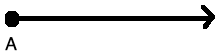Definition of an Angle

An angle is determined by rotating a ray a specific distance about its starting point. The starting position of the ray is called the initial side of the angle. After the rotation we end up with another ray and it is called the terminal side of the angle. The point where the initial and the terminal side meet is called the vertex of the angle.NOTE:  When drawing an angle, the rotation of the ray about its endpoint is usually indicated with an arc in between the initial and terminal sides.

Angle Measure

Most commonly, angles are measured in degrees. We will know that angles are measured in degrees when there is little circle in the upper right-hand corner of a number.  For example, 45o which is then pronounced 45 degrees.

Degrees can further be divided into minutes ( ' ) and seconds ( " ). That is,

1o = 60' (minutes) using the apostrophe on the computer keyboard

1' = 60" (seconds) using the quotation mark on the keyboard

Example 1:

Change 45o 14' 39" (45 degrees and 14 minutes and 39 seconds) to decimal degree form.  Round to two decimal places.

CalculateType the entire calculation into your calculator.  Do not round until you have the final answer: 45o 14' 39"45.24o

Example 2:

Change 57.68o to degrees, minutes, and seconds rounded to whole numbers.

1. The 0.68 is a fraction of a degree. Take 0.68 away from 57.68 and convert it to minutes as follows:

0.68(60') =  40.8'

2. The 0.8 from Step 1 is a fraction of a minute. Take 0.8 away from 40.8 and convert it to seconds as follows:

0.8(60") = 48"

Thus, 57.68o = 57o 40' 48"

Naming Angles

Angles can be named in several ways. In this course we are going to use three different ways.  We'll show them by using the following picture:We can place a number or letter in between the two rays, say 1, and then name the angle1 using the symbolfor angle. We pronounce this "angle 1".

We can also use the letters of points on the rays together with the vertex point. That is,ABC orCBA, either way, as long as the letter for the vertex point is in the middle.

Finally, we can use the letter for the vertex point alone as long as it is perfectly clear which angle is designated by this letter. In the picture above, it is quite clear which angle we mean when we sayB.

Sometimes Greek letters are used to represent angles. Most common are the following letters:

Theta: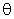Alpha:Beta: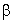Gamma: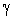Introducing Some Special Angles

Right Angles

Angles whose measure is exactly 90o.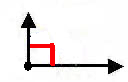Please note that the RIGHT ANGLE is usually indicated by a rectangle drawn between the terminal and initial side.

Straight Angles

Angles whose measure is exactly 180o.

Acute Angles

Angles whose measure is greater than 0o but less than 90o.

Obtuse Angles

Angles whose measure is greater than 90o but less than 180o.

Complementary Angles

Two angles are called complementary when their sum is 90o.

Supplementary Angles

Two angles are called supplementary when their sum is 180o.

Drawing and Measuring Angles

We use a protractor to draw and measure angles in degrees.  Below is a picture of one type.  Note specifically the Base, the Center, and the Scale. When measuring angles, the Center is placed at the vertex of the angle with the Base along its initial side.

Note there are two scales, one for measuring acute angles and one for measuring obtuse angles.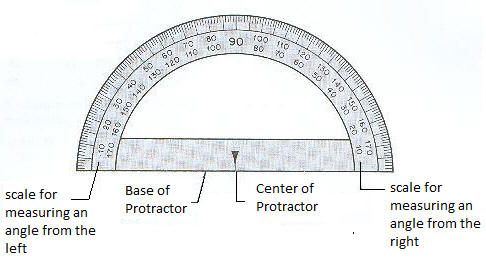Example 3:

Measure the angle O in the picture below.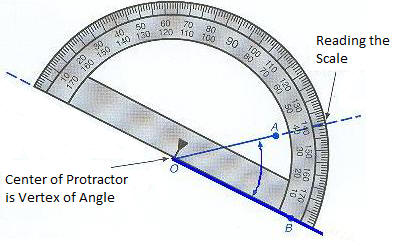We place the Base of the protractor along the terminal side of the angle with its Center at the vertex.

We read the measurement where the terminal side crosses the scale of the protractor.

The measure of angle O is 40oNOTE: Since it is an acute angle, it is certainly not 140o!!!

General Characteristics of Triangles

A triangle is a closed 2-dimensional shape with 3 sides, 3 interior angles, and 3 vertices (singular: vertex).Interior Angles of a Triangle

The interior angles of a triangle are the three angles inside the triangle. The sum of the three interior angles in a triangle is always 180o.

Sides of a Triangle

The longest side of a triangle is opposite the largest angle and vice versa. The shortest side of a triangle is opposite the smallest angle and vice versa.

Vertices (singular: vertex)

The point of intersection of any two sides of a triangle is known as a vertex. A triangle has three vertices. In the triangle above, the vertices are A, B, and C.

Introducing Several Types of Triangles

The Right Triangle

A right triangle has two sides that form a 90o angle which is also called a right angle. These two sides are called the legs of the triangle. The side opposite the right angle is called the hypotenuse.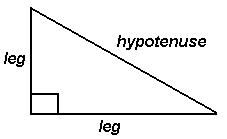Please note that the RIGHT ANGLE is usually indicated by a rectangle drawn between the legs.

The Equilateral Triangle

An equilateral triangle is a triangle with three equal sides. The three angles of an equilateral triangle are also equal.

In the triangle below,A equalsB.equalsC equals 60o.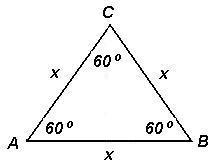The Isosceles Triangle

An isosceles triangle is a triangle with exactly two equal sides. The angles opposite the two equal sides are also equal.

In the triangle below,A equalsB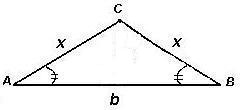The Right Isosceles Triangle

A right isosceles triangle is a triangle with exactly two equal sides. However, one of the angles is 90o. This means that the angles opposite the two equal sides each measure exactly 45o.

In the triangle below,A equalsB equals 45o.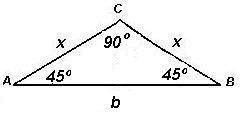The Scalene Triangle

A triangle having sides of different lengths and angles of different measure.

Acute and Obtuse Triangles

In an acute triangle all angles are greater than 0o but less than 90\o. An obtuse triangle has one angle whose measure is greater than 90o but less than 180o.